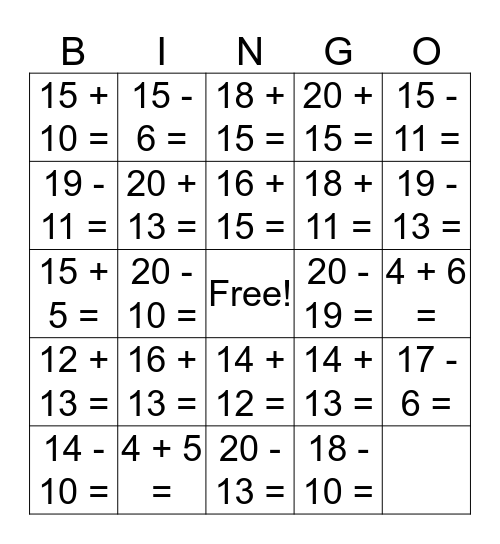# NUMBER LINE BINGOThis bingo card has a free space and 24 words: 12 + 13 =, 4 + 5 =, 14 + 12 =, 14 + 13 =, 15 - 11 =, 15 + 10 =, 15 - 6 =, 18 + 15 =, 18 + 11 =, 17 - 6 =, 19 - 11 =, 20 + 13 =, 20 -18 =, 20 - 19 =, 19 - 13 =, 14 - 10 =, 20 - 10 =, 16 + 15 =, 20 + 15 =, 4 + 6 =, 15 + 5 =, 16 + 13 =, 20 - 13 = and 18 - 10 =.

## Play Online